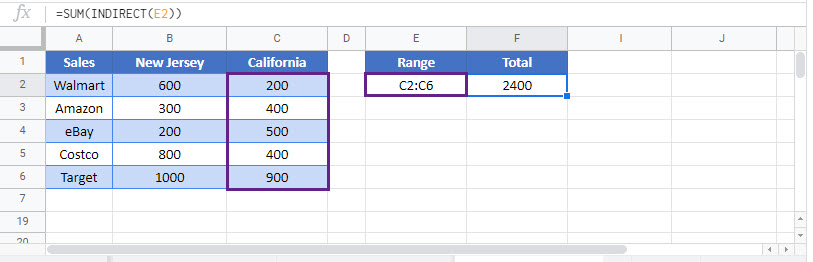# INDIRECT Sum – Excel & Google Sheets

This tutorial will teach you how to use the INDIRECT function nested in the SUM function in Excel and Google Sheets.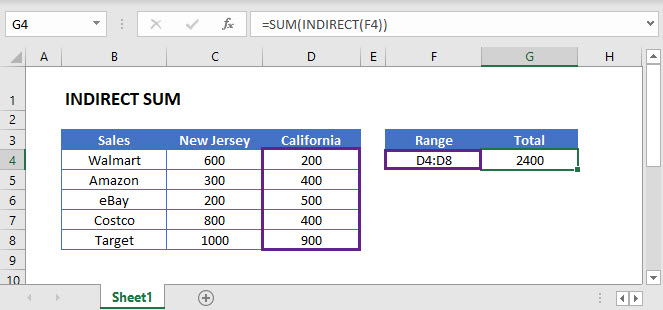## INDIRECT Sum using Cell Reference

One way to sum a dynamically referred range is by using a combination of the SUM & the INDIRECT Function to get the output. Its syntax is: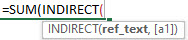This example will dynamically reference the range specified in cell F5 and sum its values.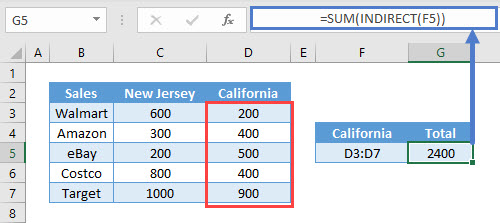In this example range address has been entered manually, accordingly, any insertion or deletion of row or column would cause the final value to change.

## INDIRECT Sum using Dynamic Sheet Reference

To sum a range dynamically referenced from another worksheet, the INDIRECT Function can be nested in the SUM Function to find the result.

This example will dynamically reference the range in worksheet ‘Texas‘ and find the sum.The INDIRECT Function along with the Concatenate Operator (&) converts the text string into a valid worksheet reference.

## INDIRECT – Volatile Function

Warning: The INDIRECT Function is volatile. This means it re-calculates every time the workbook re-calculates. If you rely on too many INDIRECT functions, it will negatively impact your workbook speed.

Alternatively, you can use other non-volatile functions. One such function is the OFFSET Function.

## Dynamic SUM using OFFSET Function

The OFFSET Function returns a reference to a range that is offset from a starting cell or range by a specified number of rows and columns. It can be nested in the SUM Function to dynamically reference a range and sum values. Its syntax is: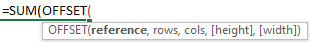Following example using the OFFSET Function will dynamically reference the range ‘D3:D7‘.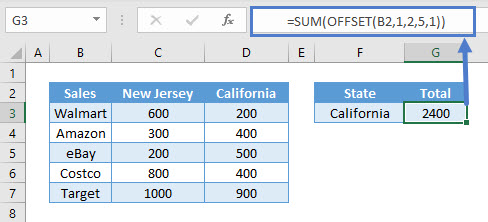Starting with cell B2, the Function offsets one (1) row and two (2) columns to obtain the range D3:D7 with a height of 5 and width 1.

If the last two arguments ‘[height]‘ and ‘[width]‘ are omitted, new reference will have the same height and width as the starting reference. Hence, alternatively a range with similar height and width can be used as the starting reference and offset the number of rows and columns to obtain the new reference.

Note: The OFFSET Function allows formulas to dynamically adjust to the data. Therefore, an addition or deletion of rows and columns would not cause the final output to change.

## INDIRECT Sum in Google Sheets

These formulas work exactly the same in Google Sheets as in Excel.